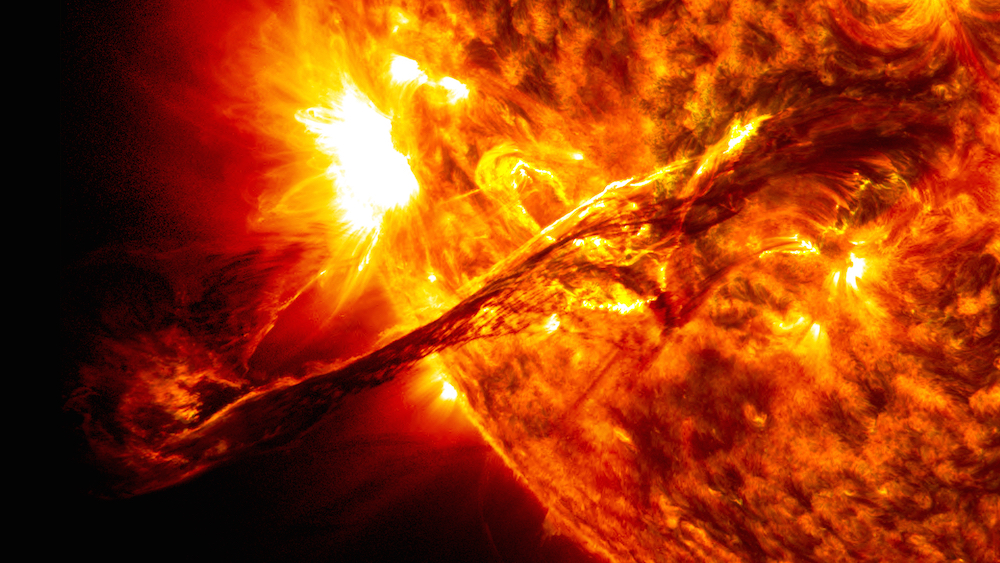# A long fallThe Earth orbits around the Sun because it has angular momentum. If we stopped the Earth in orbit and then let it fall straight towards the Sun, how long would it take to reach the sun in seconds?

Details and assumptions

• The mass of the Sun is $2 \times 10^{30}~\mbox{kg}$.
• The mass of the Earth is $6 \times 10^{24}~\mbox{kg}$.
• Newton's constant is $6.67 \times 10^{-11}~\mbox{Nm}^2/\mbox{kg}^2$.
• The Earth is $149,600,000~\mbox{km}$ from the Sun.
• You may treat the Earth and Sun as point masses.
×